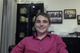Summation

To use summation, you can find sigma in the Desmos keyboard (under FUNCTIONS and then misc) or by typing "sum":If you populate the upper and lower bound, Desmos will output the summation answer.You can also put a parameter for the upper and/or lower limits:If you'd like to learn more about summation, check out the Summation page at Learn Desmos:

0 out of 0 found this helpful
Have more questions? Submit a request

•IAnthonyIsAwesome

Why can't I use a_n to represent a_0 at n=0 or any other n value?

•Anthony, just use a function a(n) instead.

My problem is that Desmos seems to quit when computing a sum without telling me that it has quit!

Edit: Nevermind, I was just being sloppy: I defined the piecewise functions I was summing over with <'s instead of <='s!

Edited by Daniel Mourad
•Tharun Dev V V

I want to sum only the odd numbers.

•Jmcinroy4813

What about a lowercase sigma?

•Welbaum Nathan

hi

•Noahrockyb

Anyone know how to input "Infinity" into the calculator?

•Shaun Errichiello

Type "infinity"

•Andrea Wahl

Any chance of Desmos actually working for infinite summations?

•Jeffrey Lu

For those of you who want only odd numbers, do the sum of 2n+1 for n=1 for as many numbers as you like.

•Flexagontnt

What can the infinity symbol be used as an input?

Article is closed for comments.